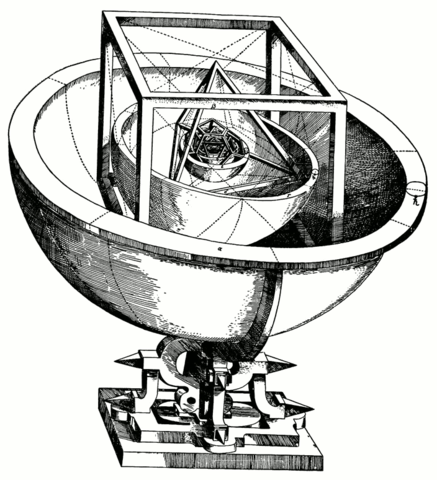# Planets and Platonic solids

Johann Kepler discovered in 1596 that the ratios of the orbits of the six planets known in his day were the same as the ratios between nested Platonic solids. Kepler was understandably quite impressed with this discovery and called it the Mysterium Cosmographicum.I heard of this in a course in the history of astronomy long ago, and have had in the back of my mind that one day I’d look into this in detail. How exactly do you fit these regular solids together? How well do the planetary ratios match the regular solid ratios?

Imagine the orbit of each planet being the equator of a spherical shell centered at the sun. The five regular solids fit snugly into the spaces between the shells. Between Mercury and Venus you can insert an octahedron. Its inradius is the distance of Mercury to the sun, and its circumradius is the distance of Venus to the sun. You can fit the other regular solids in similarly, the icosahedron between Venus and Earth, the dodecahedron between Earth and Mars, the tetrahedron between Mars and Jupiter, and the hexahedron (cube) between Jupiter and Saturn.

Here’s the data on the inradius and circumradius of each regular solid taken from Mathworld.

```    |--------------+--------------+----------|
|--------------+--------------+----------|
| octahedron   |      0.70722 |  0.40825 |
| icosahedron  |      0.95106 |  0.75576 |
| dodecahedron |      1.40126 |  1.11352 |
| tetrahedron  |      0.61237 |  0.20412 |
| hexahedron   |      0.86603 |  0.50000 |
|--------------+--------------+----------|
```

Here’s the data on average orbit radii measured in astronomical units taken from WolframAlpha.

```    |---------+----------|
| Planet  | Distance |
|---------+----------|
| Mercury |  0.39528 |
| Venus   |  0.72335 |
| Earth   |  1.00000 |
| Mars    |  1.53031 |
| Jupiter |  5.20946 |
| Saturn  |  9.55105 |
|---------+----------|
```

So how well does Kepler’s pattern hold? In the table below, “Planet ratio” is the radius ratios of Venus to Mercury, Earth to Venus, etc. “Solid ratio” is the circumradius to inradius ratio of the regular solids in the order given above.

```    |--------------+-------------|
| Planet ratio | Solid ratio |
|--------------+-------------|
|      1.82997 |     1.73205 |
|      1.38246 |     1.25841 |
|      1.53031 |     1.25841 |
|      3.40419 |     3.00000 |
|      1.83340 |     1.73205 |
|--------------+-------------|
```

Not a bad fit, but not great either. I’ve heard that the fit was better given the data available to Kepler at the time; if Kepler had had more accurate data, he might not have come up with his Mysterium Cosmographicum.

By the way, notice some repetition in the solid ratios. The implied equalities are exact. The icosahedron and dodecahedron have the same ratio of circumradius to inradius because they are dual polyhedra. The same is true for the cube and the octahedron. Also, the ratio of 3 for the tetrahedron is exact.

Update: What if Kepler had known about more planets? The next planet ratios in our table above would be 2.01, 1.57, and 1.35. None of these is close to any of the solid ratios.

## 4 thoughts on “Planets and Platonic solids”

1. Quantum Mechanic

At first blush it seems awkward to use inradius, which is based on unit polygon side length, to some normalized radius, for the purposes of nesting. Instead it seems more useful to normalize the inradius to 1, and compute the resulting circumradius ratio.

2. Tomas Singliar

There may actually be some merit to the hypothesis!

We know orbital resonance (orbit times tending towards small integer ratios) is a real phenomenon. In such cases, here is a only a small number of points on the orbits where syzygia can occur. These could well map to vertices of a polyhedron.

Unfortunately for Kepler’s hypothesis, the major planets of our solar system are not so bound.

I would be amazed by the beautiful structure, but not be entirely surprised, if together with the square and cube roots provided by Kepler’s third law the inradii and circumradii worked out to be the solution for some integer ratios or orbit times.

3. Billy Krier

Cylindrical ring currents using Bessel functions are a better fit than the upgraded Bode’s law; I believe from watching Donald Scott Electric Universe presentation on Cosmic Power Lines a while ago. Bessel functions arise in 3d wave equations like drums as well. So, there is still harmonic/musical beauty there. But again, not quite a perfect match.